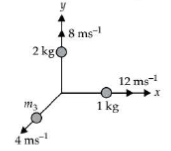Third law
Question

# An explosion breaks a rock into three parts in a horizontal plane. Two of them go off at right angles to each other. The first part of mass 1 kg moves with a speed of$12\text{\hspace{0.17em}\hspace{0.17em}}m{s}^{-1}$and the second part of mass 2 kg moves with $8\text{\hspace{0.17em}\hspace{0.17em}}m{s}^{-1}$speed. If the third part flies off with$4\text{\hspace{0.17em}\hspace{0.17em}}m{s}^{-1}$speed, then its mass is

Moderate
Solution

## The situation is as shown in the figure.According to law of conservation of linear momentum

Get Instant Solutions